Next: 13.2.4.6 CMY Conversion Up: 13.2.4 The Color Matrix Previous: 13.2.4.4 Modifying Saturation   Contents

13.2.4.5 Hue Rotation

Changing the hue of a color may be accomplished by loading a rotation about the gray vector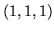. This operation may be performed in one step using the glRotate() command. The matrix may also be constructed via the following steps :

49.
50.
Rotate such that the gray vector maps onto the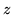axis using the glRotate() command.
51.
Rotate about theaxis to adjust the hue (glRotate<degrees>, 0, 0, 1(<degrees>, 0, 0, 1)).
52.
Rotate the gray vector back into position.
Unfortunately, a naive application of glRotate() will not preserve the luminance of the image. To avoid this problem, you must make sure that areas of constant luminance map to planes perpendicular to theaxis when you perform the hue rotation. Recalling that the luminance of a vector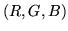is equal to: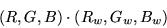you realize the plane of constant luminance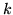is defined by: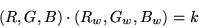Therefore, the vector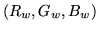is perpendicular to planes of constant luminance. The algorithm for matrix construction becomes the following :
53.
54.
Apply a rotation matrix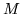such that the gray vectormaps onto the positiveaxis.
55.
Compute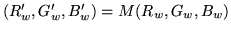. Apply a skew transform which maps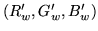to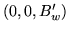. This matrix is: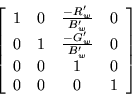56.
Rotate about theaxis to adjust the hue.
57.
Apply the inverse of the shear matrix.
58.
Apply the inverse of the rotation matrix.
It is possible to compute a single matrix as a function of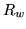,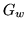,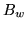, and the degrees of rotation which performs this operation.Next: 13.2.4.6 CMY Conversion Up: 13.2.4 The Color Matrix Previous: 13.2.4.4 Modifying Saturation   Contents
2001-01-10